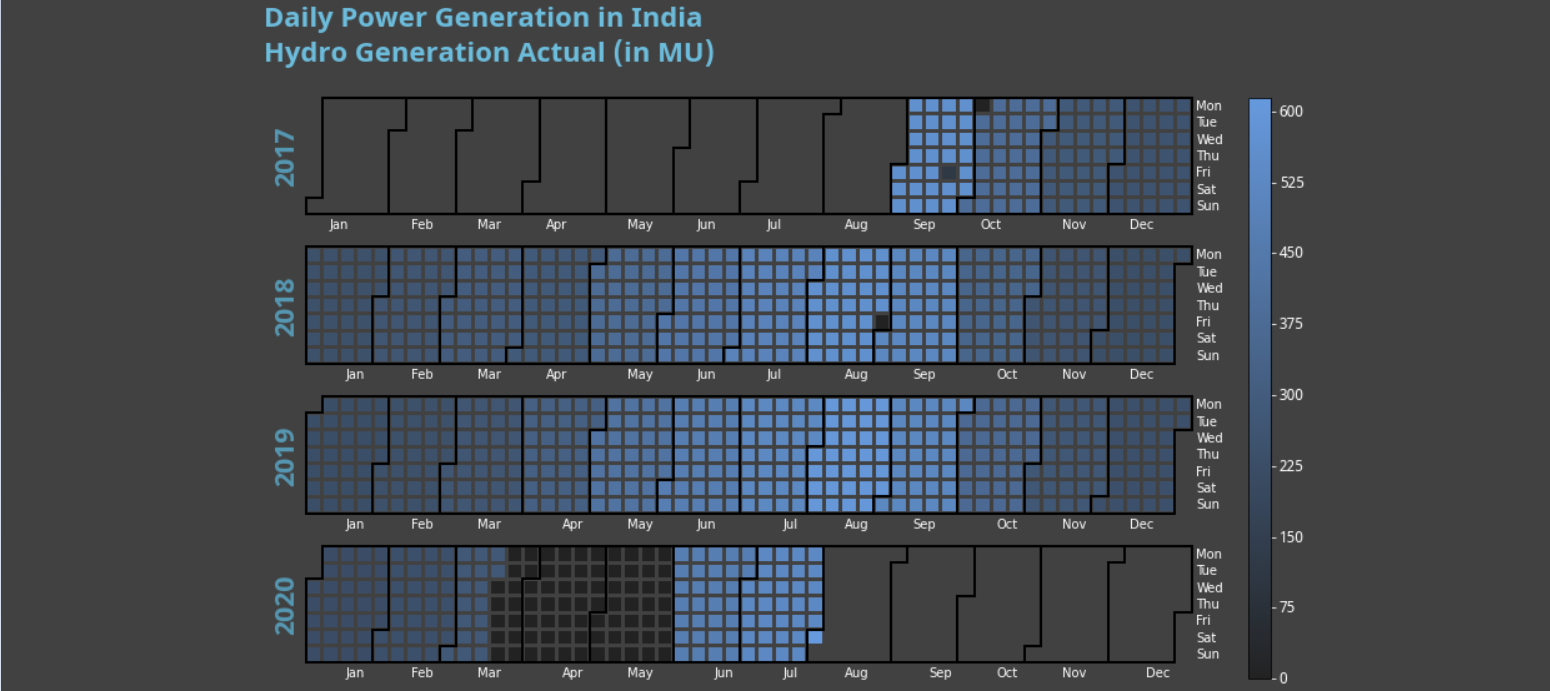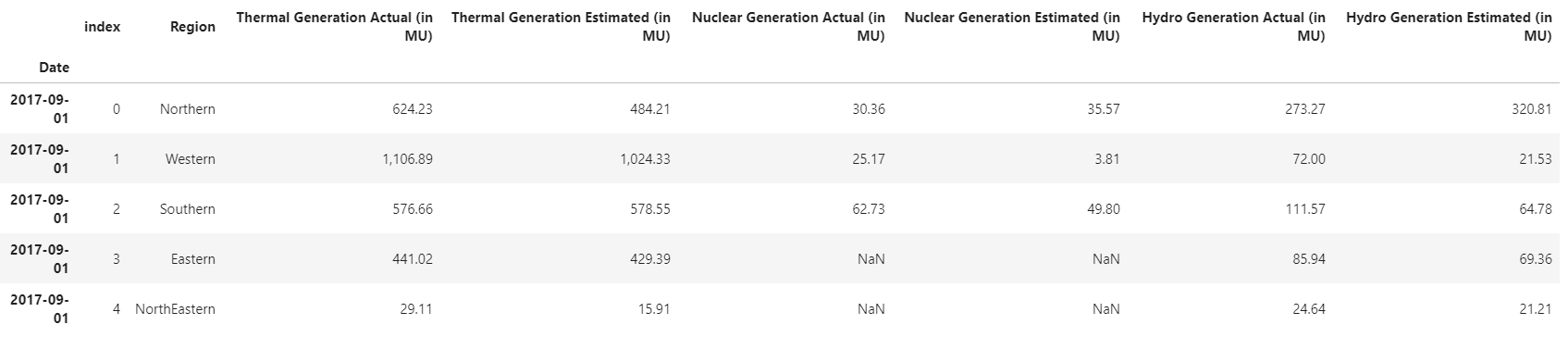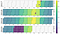# Calendar Heatmaps with Python’s Calplot

## A quick guide on plotting and customizing calendar heatmaps with PythonCalendar Heatmap — Image by the author

Few visualizations are so intuitive and insightful as calendar heatmaps are at presenting time series data. It could be because they combine two very familiar visualizations, color coding and calendars.

You probably know someone who has a planner or a calendar full of notes; some may use brighter colors to mark tasks requiring more attention, or maybe they color-code everything by category.

The concept of calendar heatmaps is very similar. We encode a variable with color and plot them in a calendar format to understand its relationship with time.

This tutorial will explore a convenient package called Calplot to draw our calendar heatmaps quickly.

## Data preparation

We can start by loading our dataset into Pandas, ensuring the date field is in the correct format and set it as the data frame index.

`import pandas as pd# kaggle.com/navinmundhra/daily-power-generation-in-india-20172020df = pd.read_csv('data/dailyPowerGeneration.csv')df['Date'] = pd.to_datetime(df.Date, yearfirst=True)df.set_index('Date', inplace=True)df.head()`Data Frame — Image by the author

## The Basics

Calplot is very straightforward, and with a single line of code, we can get a pretty nice-looking chart.

`import pandas as pdimport calplot # https://github.com/tomkwok/calplot# kaggle.com/navinmundhra/daily-power-generation-in-india-20172020df = pd.read_csv('data/dailyPowerGeneration.csv')df['Date'] = pd.to_datetime(df.Date, yearfirst=True)df.set_index('Date', inplace=True)# plotcol = 'Hydro Generation Actual (in MU)'calplot.calplot(df[col], how='sum')`Calplot’s Default Chart — Image by the author

We passed the data frame, and an argument called How. This second parameter defines what function will be used to aggregate the data. Our chart will represent the time series in days, so if we have more than one row per day, Calplot will know how to deal with it.

Calplot uses Pandas .agg for this aggregation — That means we can use Numpy functions such as mean, std, var, prod, or even our own.

## Customizing

We can use many parameters to customize our plot; we can add a title, change the colors of the polygons, the lines, and the inside of empty cells.

We can also change the color map, and since Calplot is built on top of Matplotlib, we can even use Seaborn.

`import pandas as pdimport calplot # https://github.com/tomkwok/calplotimport matplotlib.pyplot as pltimport seaborn as sb# kaggle.com/navinmundhra/daily-power-generation-in-india-20172020df = pd.read_csv('data/dailyPowerGeneration.csv')df['Date'] = pd.to_datetime(df.Date, yearfirst=True)df.set_index('Date', inplace=True)# variablescol = 'Hydro Generation Actual (in MU)'title = 'Daily Power Generation in India\nHydro Generation Actual (in MU)\n'cmap = sb.dark_palette("#69d", as_cmap=True)# plotcalplot.calplot(df[col], how='sum',                 suptitle=title, cmap=cmap,                linecolor='w', linewidth=2,                 fillcolor='w', edgecolor='black')plt.show()`

We can also change many other properties of our chart with kwargs. Calplot makes those available for many parts of our graph, such as the suptitle, subplots, figure, year label, and grid spec.

`import pandas as pdimport calplot # https://github.com/tomkwok/calplotimport matplotlib.pyplot as pltfrom matplotlib import font_manager as fmimport seaborn as sb# kaggle.com/navinmundhra/daily-power-generation-in-india-20172020df = pd.read_csv('data/dailyPowerGeneration.csv')df['Date'] = pd.to_datetime(df.Date, yearfirst=True)df.set_index('Date', inplace=True)col = 'Hydro Generation Actual (in MU)'title = 'Daily Power Generation in India\nHydro Generation Actual (in MU)\n'facecolor = '#414141'font_path = "fonts/NotoSans-Bold.ttf"fontproperties = fm.FontProperties(fname=font_path, size=22)yearlabel_kws = dict(fontproperties=fontproperties                     ,color='#5698B3'                     ,ha='center')suptitle_kws = dict(fontproperties=fontproperties                     ,color='#6CBCDC'                     ,ha='left'                     ,y=1.125                     ,x=-0.025)fig_kws = dict(facecolor=facecolor)subplot_kws = dict(facecolor=facecolor)cmap = sb.dark_palette("#69d", as_cmap=True)calplot.calplot(df[col], how='sum',                 suptitle=title, suptitle_kws=suptitle_kws,                yearlabel_kws=yearlabel_kws, fig_kws=fig_kws,                subplot_kws = subplot_kws, linecolor=facecolor,                edgecolor='black', fillcolor=facecolor,                 linewidth=2, cmap=cmap)plt.show()`

All other arguments are passed to Calplot’s method `yearplot`.

We could, for example, pass a formating string to `textformat='{:.0f}'` which will print the values for each day.

## Going Further

Even if there isn’t an argument for what you want, Calplot returns Matplotlib’s figure and axes, and we can use those to change whatever we want.

`import pandas as pdimport numpy as npimport calplot # https://github.com/tomkwok/calplotimport matplotlib.pyplot as pltfrom matplotlib import font_manager as fmimport seaborn as sbimport calendar# # kaggle.com/navinmundhra/daily-power-generation-in-india-20172020df = pd.read_csv('data/dailyPowerGeneration.csv')df['Date'] = pd.to_datetime(df.Date, yearfirst=True)df.set_index('Date', inplace=True)col = 'Hydro Generation Actual (in MU)'title = 'Daily Power Generation in India\nHydro Generation Actual (in MU)\n'facecolor = '#414141'font_path = "fonts/NotoSans-Bold.ttf"fontproperties = fm.FontProperties(fname=font_path, size=22)yearlabel_kws = dict(fontproperties=fontproperties                     ,color='#5698B3'                     ,ha='center')suptitle_kws = dict(fontproperties=fontproperties                     ,color='#6CBCDC'                     ,ha='left'                     ,y=1.125                     ,x=-0.025)fig_kws = dict(facecolor=facecolor)subplot_kws = dict(facecolor=facecolor)cmap = sb.dark_palette("#69d", as_cmap=True) fig, axes = calplot.calplot(df[col],                            how='sum',                            suptitle=title,                            suptitle_kws=suptitle_kws,                            yearlabel_kws=yearlabel_kws,                            fig_kws=fig_kws,                            subplot_kws = subplot_kws,                            linecolor=facecolor,                             edgecolor='black',                            fillcolor=facecolor,                             linewidth=2, cmap=cmap)for ax in axes:    ax.set_xticklabels(calendar.month_abbr[1:], color='w')    ax.set_yticklabels(calendar.day_abbr[:], color='w')plt.show()`

The package is straightforward, with about 350 lines of code where more than 100 are comments; it’s easy to change or adapt it to our needs.

For example, I couldn’t find a way to change the color-bar ticks. But it’s no trouble to make Calplot return the cbar.

`# calplot.py# After line 336  cb = None  if colorbar:    if tight_layout:      stitle_kws.update({'x': 0.425, 'y': 1.03})    if len(years) == 1:      cb = fig.colorbar(axes.get_children(),                        ax=axes.ravel().tolist(),                        orientation='vertical')    else:      fig.subplots_adjust(right=0.8)      cax = fig.add_axes([0.85, 0.025, 0.02, 0.95])      cb = fig.colorbar(axes.get_children(), cax=cax,                        orientation='vertical')  stitle_kws.update(suptitle_kws)  plt.suptitle(suptitle, **stitle_kws)  return fig, axes, cb`

We can then access the color bar and make the changes we want.

`import pandas as pdimport numpy as npimport calplot # https://github.com/tomkwok/calplotimport matplotlib.pyplot as pltfrom matplotlib import font_manager as fmimport seaborn as sbimport calendar# # kaggle.com/navinmundhra/daily-power-generation-in-india-20172020df = pd.read_csv('data/dailyPowerGeneration.csv')df['Date'] = pd.to_datetime(df.Date, yearfirst=True)df.set_index('Date', inplace=True)col = 'Hydro Generation Actual (in MU)'title = 'Daily Power Generation in India\nHydro Generation Actual (in MU)\n'facecolor = '#414141'font_path = "fonts/NotoSans-Bold.ttf"font_path_cbar = "fonts/NotoSans-Regular.ttf"fontproperties = fm.FontProperties(fname=font_path, size=22)fontproperties_cbar = fm.FontProperties(fname=font_path_cbar, size=12)yearlabel_kws = dict(fontproperties=fontproperties                     ,color='#5698B3'                     ,ha='center')suptitle_kws = dict(fontproperties=fontproperties                     ,color='#6CBCDC'                     ,ha='left'                     ,y=1.125                     ,x=-0.025)fig_kws = dict(facecolor=facecolor)subplot_kws = dict(facecolor=facecolor)cmap = sb.dark_palette("#69d", as_cmap=True) fig, axes, cbar = calplot.calplot(df[col],                            how='sum',                            suptitle=title,                            suptitle_kws=suptitle_kws,                            yearlabel_kws=yearlabel_kws,                            fig_kws=fig_kws,                            subplot_kws = subplot_kws,                            linecolor=facecolor,                             edgecolor='black',                            fillcolor=facecolor,                             linewidth=2, cmap=cmap)for ax in axes:    ax.set_xticklabels(calendar.month_abbr[1:], color='w')    ax.set_yticklabels(calendar.day_abbr[:], color='w')cbar.set_ticks(np.arange(0,601,75))cbar.ax.yaxis.set_tick_params(color='w')plt.setp(plt.getp(cbar.ax.axes, 'yticklabels'), color='w', fontproperties=fontproperties_cbar, va='center')plt.show()`

With a calendar heatmap, we can see the patterns and extract insights.

Even in the first example we built, we could see more energy produced in the summer. We can note outliers in September and October 2017 and August 2018, and there‘s a considerable period in 2020 without production that we could investigate. Maybe it’s just missing data, or perhaps it has something to do with Covid.

Thanks for reading. I hope you enjoyed it!

Data visualization enthusiast